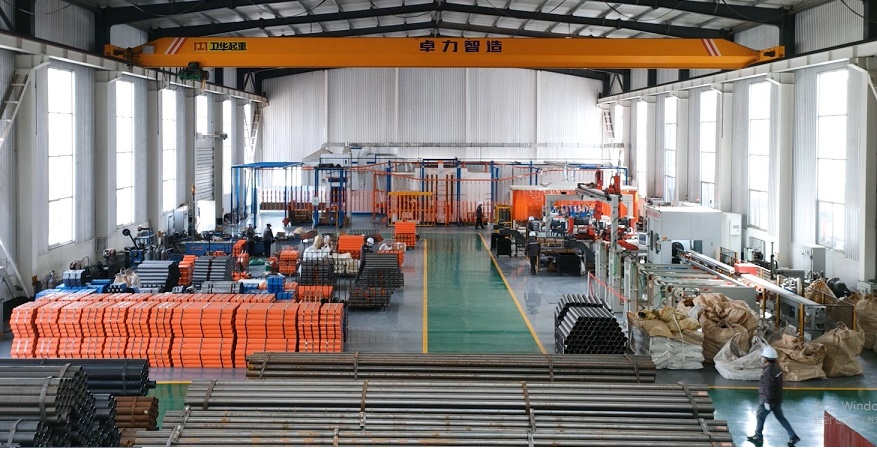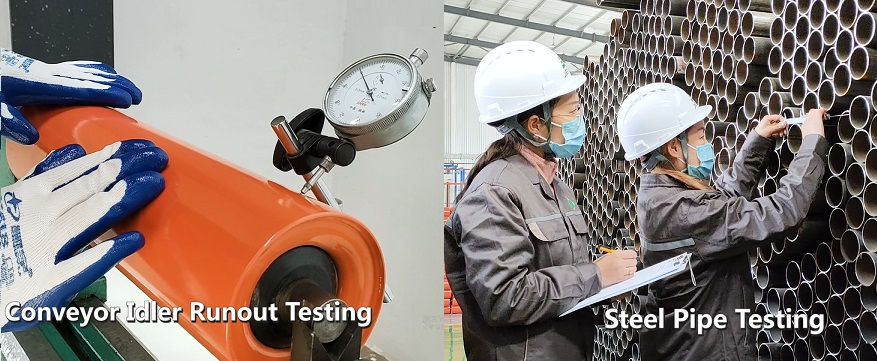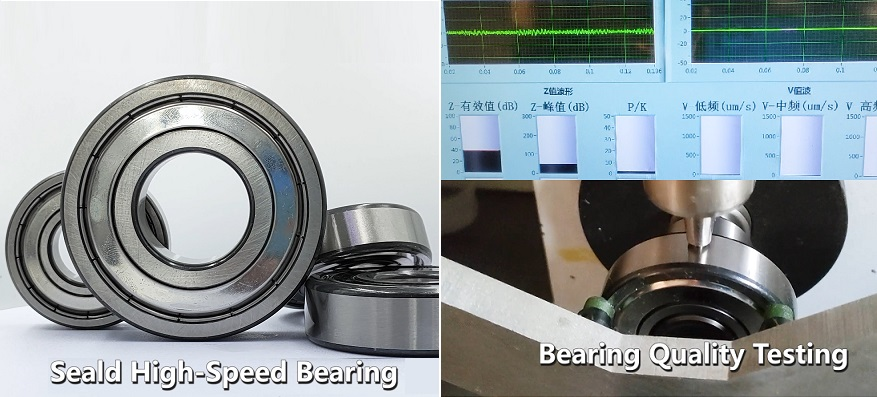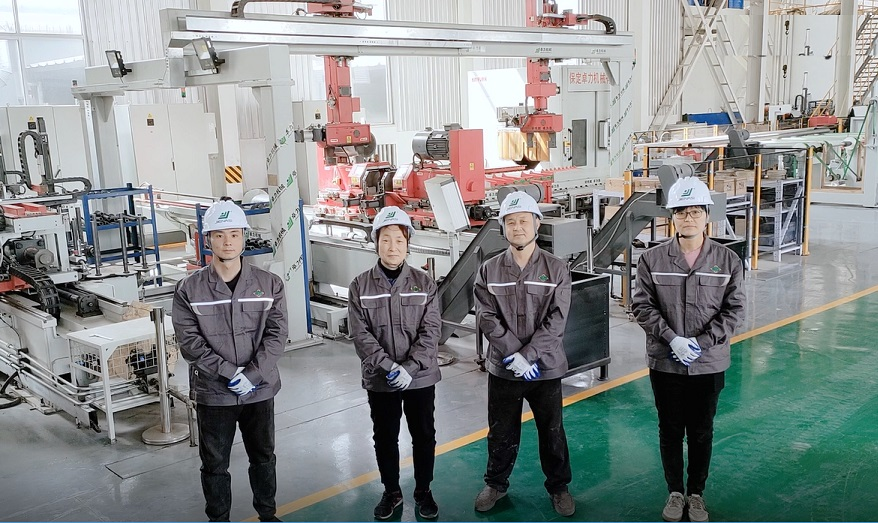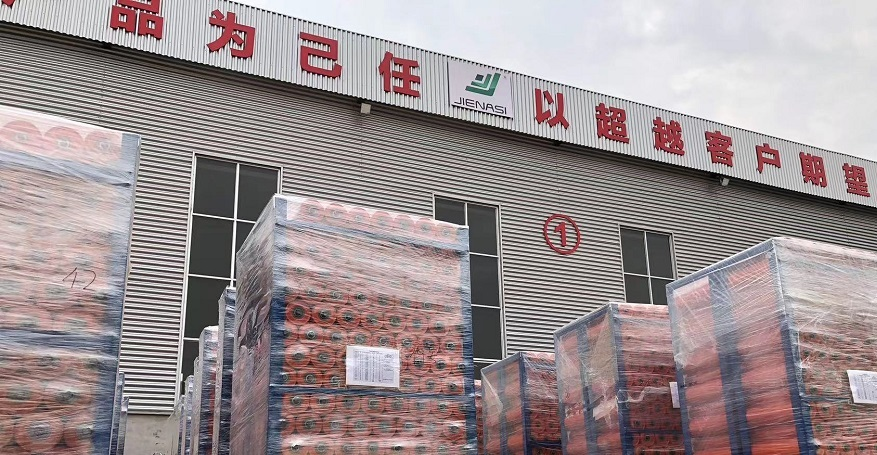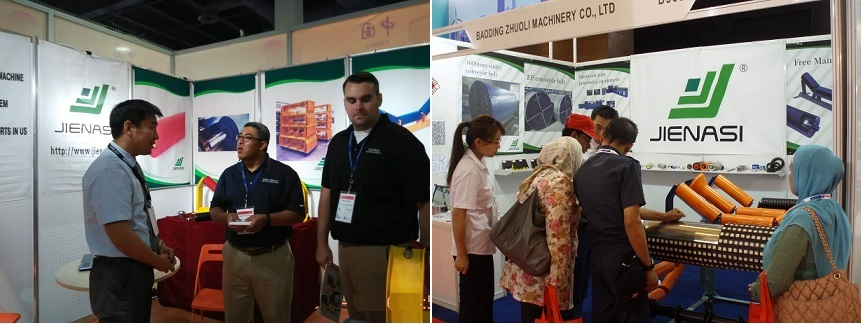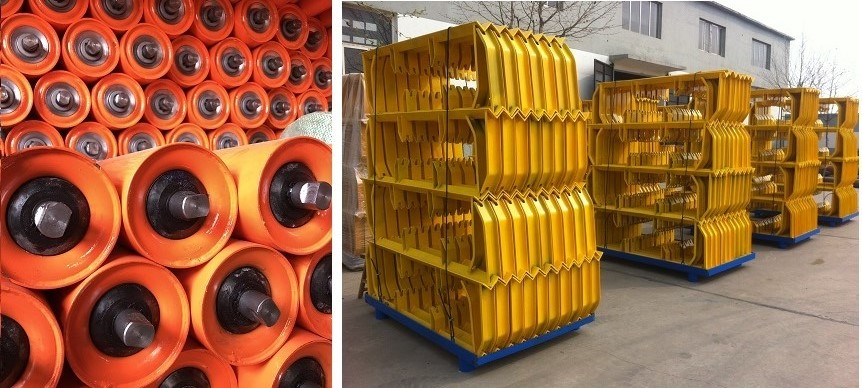# What is Conveyor Idler static and Dynamic Load?-JIENASI Conveyor Idler

Conveyor idlers play a crucial role in supporting the conveyor belt and transporting materials. Correctly calculating the static and dynamic loads that act on the idlers is essential for selecting suitable idlers and estimating their service life. This article will provide a detailed overview of various aspects related to the calculation of conveyor idler loads, including types and dimensions of conveyor idlers, characteristics of conveyed materials and conveyor belts, operating conditions and scenarios, axial load capacity, radial load capacity, bearing friction, and dynamic effects.

1.Types and Dimensions of Conveyor Idlers
Conveyor idlers come in various types, such as trough idlers, flat idlers, self-aligning idlers, and more. Depending on the characteristics of the conveyor belt and the operating environment, it's essential to choose the appropriate type and size of conveyor idlers. The dimensions of the idlers primarily depend on the width of the supported conveyor belt and the load it will bear. Generally, larger idler diameters correspond to higher load-bearing capacities.

2.Characteristics of Conveyed Materials and Conveyor Belts
Calculating conveyor idler loads necessitates considering the characteristics of both conveyed materials and conveyor belts. Material properties, including density, particle size, and humidity, influence the distribution of loads on the conveyor belt. Conveyor belt characteristics, such as material composition, tension, and elasticity, also impact the idler loads. Accurate load calculations for idlers can be achieved by measuring and analyzing these parameters.

3.Operating Conditions and Scenarios
Calculations for conveyor idler loads should also account for operating conditions and scenarios. Factors like conveyor speed, load capacity, and incline angle affect the idler loads. Harsh working environments, such as high temperature, humidity, or corrosive conditions, also influence idler loads. By considering real-world operating conditions and scenarios, more precise predictions of idler lifespan and performance can be made.

The axial load capacity refers to the load that a conveyor idler bears in the direction of its movement along the conveyor belt. The formula for calculating this load is:

F_ax = (q_b + q_d) * g * cos(θ)

Where, F_ax represents the axial load capacity, q_b is the mass of the conveyed material on the belt, q_d is the mass of the conveyor belt, g is the acceleration due to gravity, and θ is the inclination angle of the conveyor belt.

The radial load capacity refers to the load that a conveyor idler bears vertically to the direction of the conveyor belt. The formula for calculating this load is:

F_rx = q_b * g * sin(θ)

Where, F_rx denotes the radial load capacity, and the other parameters are the same as mentioned above.

6.Bearing Friction
Bearing friction is also a significant factor in conveyor idler load calculations. The magnitude of bearing friction is influenced by factors such as bearing type, lubrication, bearing clearance, etc. In general, bearing friction can be calculated using the following formula:

F_fr = f * F_ax

Where, F_fr stands for bearing friction, f represents the coefficient of friction, and the other parameters remain the same.

In static load calculation, the weight of the conveyor material and the conveyor belt generates a forward pressure on the idlers. In addition, the tension of the conveyor belt must be considered in the bending section, particularly in the concave and convex arcs. The force analysis of grooved conveyor idlers mainly involves the load distribution on the three idlers, particularly in the convex arc segment. Currently, most calculations assume that the intermediate idler bears the most stress, which is estimated at 70% of the weight of the material and 40% of the weight of the conveyor belt. However, the bearings of the two idlers after the side idlers are loaded are not equal, resulting in a relatively large axial force.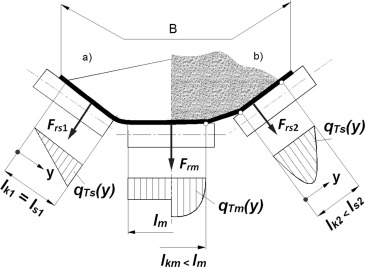Dynamic load must also be considered when selecting conveyor idlers. Dynamic load is generated by various factors, including the eccentric rotation of the idler shell, eccentric rotation of the idler and the conveyor belt, impact of the conveyor belt and material during movement, lateral bending vibration of the conveyor belt against the idler, tension of the conveyor belt on the transition section and curved section idlers, deformation of the material and the conveyor belt when passing the idler (especially when the material contains large blocks), and impact load of the idler where the material is dropped by the falling material.

For example, the dynamic load caused by the eccentric rotation of the idler shell can be calculated using the formula F=0.45Mw, where F is the dynamic load (kN), and Mw is the unbalanced mass moment of the idler shell (kg m^2). The dynamic load caused by the impact of the conveyor belt and material can be estimated using the formula F=Kvma, where Kv is the impact coefficient, ma is the mass of the material per unit length (kg/m), and a is the acceleration of the material (m/s^2).

In summary, accurately calculating the static and dynamic loads acting on conveyor idlers is critical for ensuring the safe and efficient operation of conveyor systems.